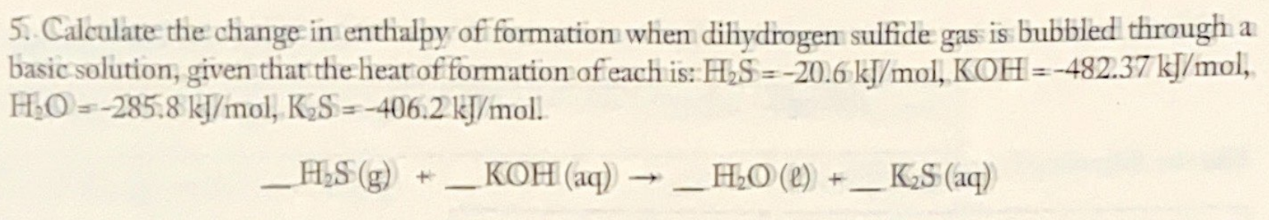# 5. Calculate the change in enthalpy of formation when dihydrogen sulfide gas is bubbled through a basic solution, given that the heat of formation of each is: H2S = -20.6 kJ/mol, KOH = -482.37 kJ/mol, H2O = -285.8 kJ/mol, K2S = -406.2 kJ/mol. __H2S (g) + __ KOH (aq) → __ H2O (l) + __ K2S(aq)